# 应用视觉效果

## 应用图层混合

1. 内容窗格中，单击图层标题以将其高亮显示。
2. 在图层的上下文选项卡下，单击外观选项卡。
3. 效果组中，单击图层混合下拉菜单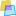选择要应用于图层的混合模式。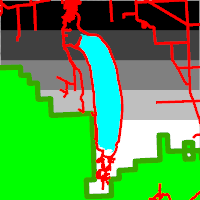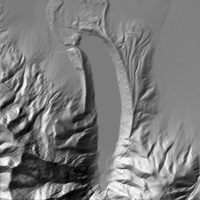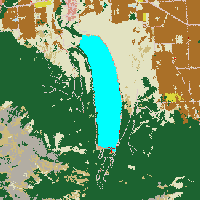### 加亮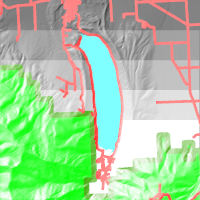= 1 - (1 - a) * (1 - b)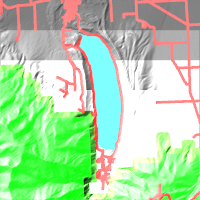= a / (1 - b)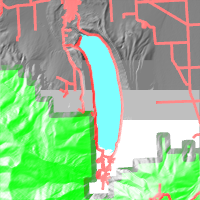= max(a, b)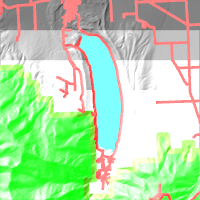= a + b

### 加深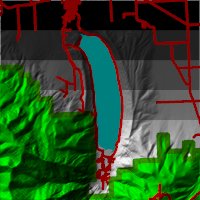=(a * b)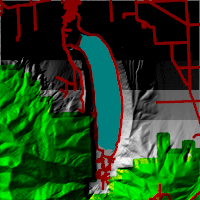= 1 - (1 - a) / b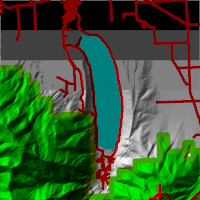= min(a, b)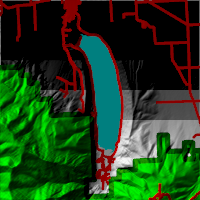= a + b - 1

### Comparison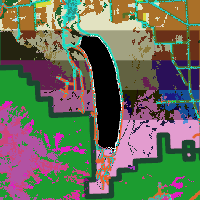= abs(a - b)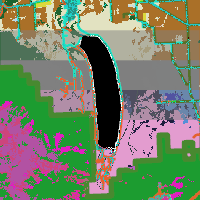= 0.5 - 2*(a - 0.5)*(b - 0.5)

### 发散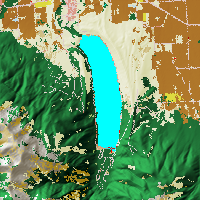= 2ab（如果 a < 0.5）；否则为 (1 - 2 * (1 - a) * (1 - b))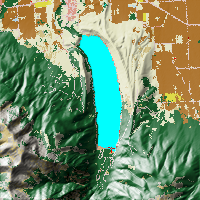= 2ab（如果 b < 0.5）；否则为 (1 - 2 * (1 - a) * (1 - b))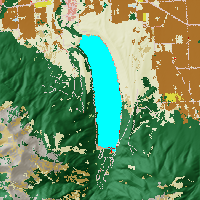(b > 0.5) * (1 - (1 - a) * (1-(b-0.5))) + (b <= 0.5) * (a * (b+0.5))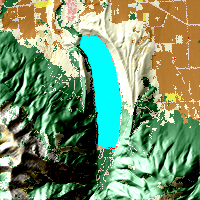(b > 0.5) * (a + 2*(b - 0.5)) + (b <= 0.5) * (a + 2*b - 1)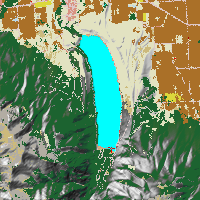(b > 0.5) * (max(a,2*(b - 0.5))) + (b <= 0.5) * (min(a,2*b)))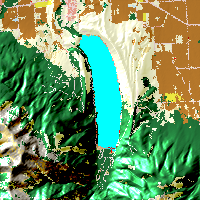(b > 0.5) * (1 - (1-a) / (2*(b - 0.5))) + (b <= 0.5) * (a / (1-2*b))

### 颜色组合模式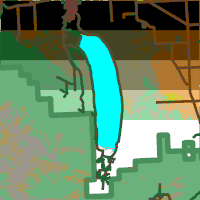= 色调(a) + 饱和度(a) + 亮度(b)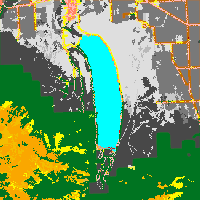= 色调(a) + 饱和度(b) + 亮度(a)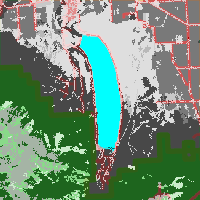= 色调(b) + 饱和度(a) + 亮度(a)

Color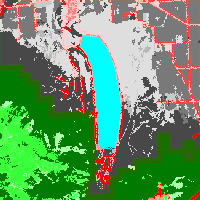= 色调(b) + 饱和度(b) + 亮度(a)

## 应用要素混合1. 内容窗格中，单击要素图层标题以将其高亮显示。

要素图层上下文选项卡将显示在功能区中。

2. 要素图层下，单击外观选项卡。
3. 效果组中，单击要素混合下拉菜单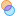选择要应用于图层要素的混合模式。